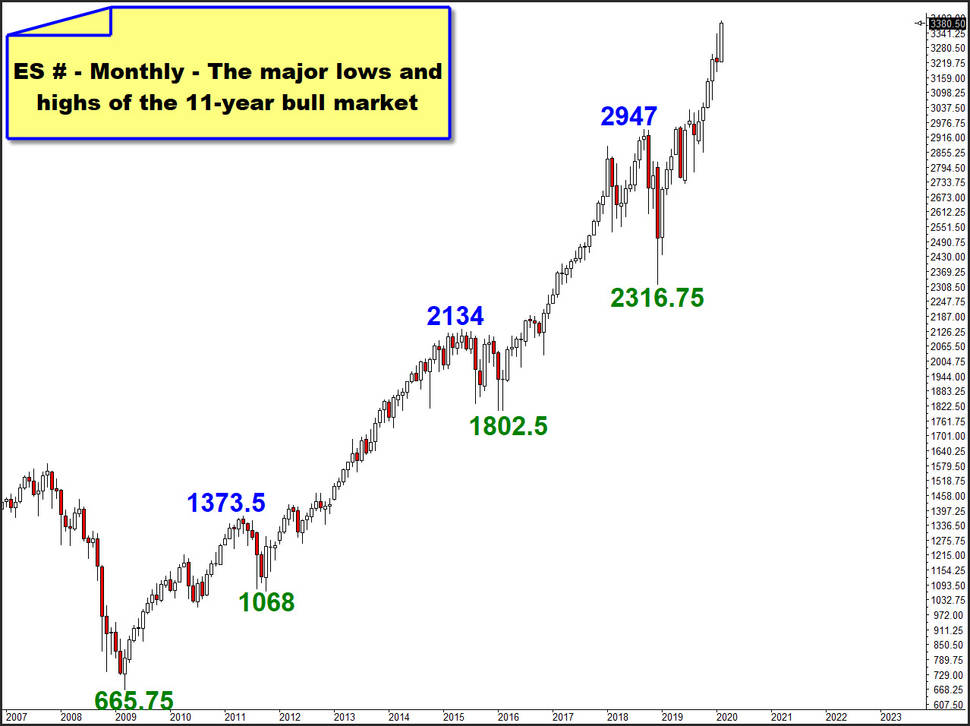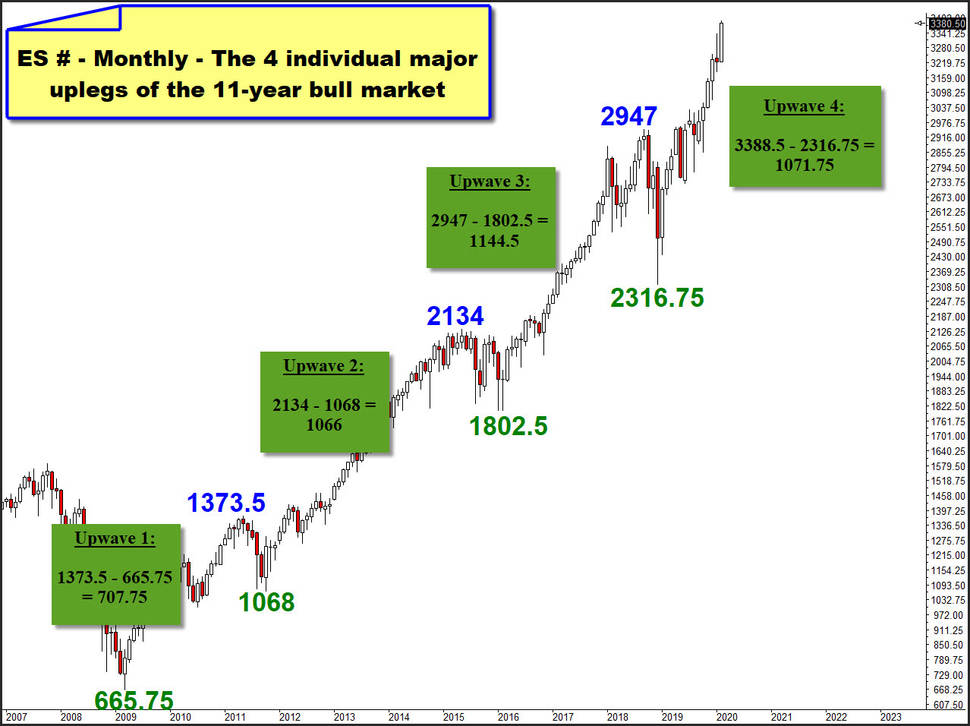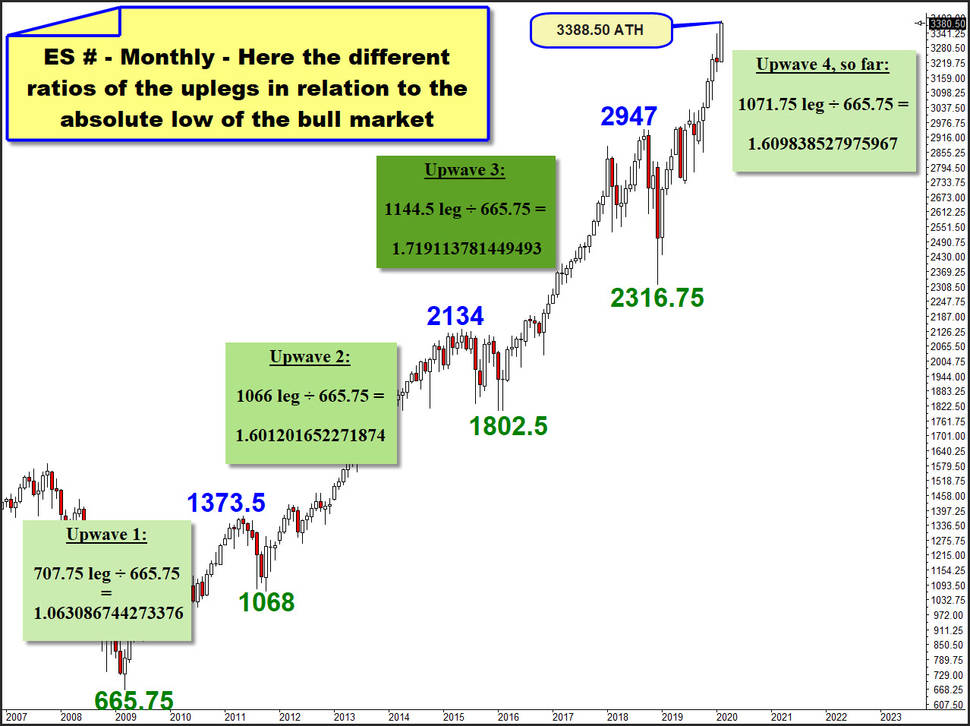# Now the S&P 500 bull is at the Golden Ratio - again!

As of Friday, the US stock market rally, which began at the Christmas lows of 2018, reached the most prominent and most noted Fibonacci ratio namely the Golden Ratio what is the mathematical constant of 1.6180339...

The Golden Ratio is closely associated with the Fibonacci sequence 1, 1, 2, 3, 5, 8, 13, 21, 34, ... Among many other things, the ratios of successive Fibonacci numbers converge to phi. For example:

... 5/3=1.666666

8/5=1.600000

13/8=1.625000

21/13=1.615385

34/21=1.619048

55/34=1.617647

89/55=1.6181821

144/89=1.617978 ...

Fibonacci price projections or Fibonacci expansions is a support and resistance indicator that uses Fibonacci ratios to analyze price movements and identify potential support and resistance levels. The proportions used in Fibonacci projection studies are based on the mathematical relationships, expressed as ratios, between the numbers in the Fibonacci summation series.The key Fibonacci projection levels are found by performing mathematical operations on the numbers in the Fibonacci summation series, and on the results of those operations. The same levels used in Fibonacci price extensions are used in Fibonacci projections:

The most known and most respected mass movement key was and for ever will be the ratio of 161.8%, which is the famous Golden Ratio. The Golden Ration is found by dividing a number in the sequence by the number that precedes it. For example: 21 ÷ 13 = 1.615385, 34 ÷ 21 = 1.619048 or 55 ÷ 34 = 1.617647, etc.

Always when the Golden Ratio becomes visible in the charts it indicates the possible end of the existing trend or the beginning of a price reversion event.

Already 2 earlier bull market upwaves of the S&P 500 emini (ES #) were based on the Golden Ratio. The last two important bull market legs, which I name upwave 2 and upwave 3 in the monthly charts below. At the moment we are in the 4th major upward wave of the bull market, which started at the lows of 2009 and on Friday made next absolute bull market high at 3388.50 pts:And next, we bring the individual successes of upwaves 1-4 in relation to the evil low 665.75 pts (666), the price where this bull market started 2009, what is the most important price of the current MILLENNIUM and CENTURY so far.1373.5 – 665.75 = 707.75 advance of upwave 1

707.75 ÷ 665.75 = 1.063086744273376‬

2134 – 1068 = 1066 pts rise for upwave 2

1066 ÷ 665.75 = 1.601201652271874‬

==> Upleg 2 was fully oriented to the Golden Ratio! Afterwards longest correction of the 11-year bull started.

2947 – 1802.5 = 1144.5 pts gain within upwave 3

1144.5 ÷ 665.75 = 1.719113781449493

==> Upwave 3 ratio is just above the perfect Golden Ratio, followed by sharpest and deepest correction leg of bull what bottomed at 2316.75 pts year 2018 Xmas Low.

Friday printed alltime-high at 3388.50 pts, what results in this ratio-calculation for upwave 4, so far:

3388.5 – 2316.75 = 1071.75 upwave 4

1071.75 ÷ 665.75 = 1.609838527975967‬

==> and is thus in exact sync to the upwave 2 advance and the Golden Ratio.

As markets have inertia and know about the 1.6180339 phy – especially the Algos ... -, it may well be that considerable resistance has been reached, which could soon lead to a major trend change!

Since forming an important top is usually a somewhat persistent process, we should not expect the markets to turn on a dime visibly soon. In my opinion - forewarned is forearmed! -, it is definitely no bad idea to start now with the closing of larger longterm and medium-term oriented equity longs.

For us, however, it must also be clear and expectable that it can continue to rise so unnervingly slowly, because the market may want to orientate itself to the advances of upwave 3. Then would be:

665.75 * 1.719113781449493 = 1144.5 + 2316.75 low = a 3.461.25‬ ES # pts would be next higher major target price when we take the evil low into account!

Be prepared!

Eduard Altmann